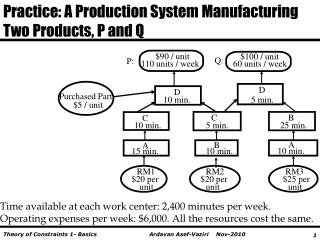DownloadDownload PresentationPurchased Part

Purchased Part

Download PresentationPurchased Part

- - - - - - - - - - - - - - - - - - - - - - - - - - - E N D - - - - - - - - - - - - - - - - - - - - - - - - - - -
Presentation Transcript

1. Practice: A Production System Manufacturing Two Products, P and Q \$90 / unit \$100 / unit Q: P: 60 units / week 110 units / week D D Purchased Part 10 min. 5 min. \$5 / unit C B C 10 min. 5 min. 25 min. B A A 15 min. 10 min. 10 min. RM1 RM2 RM3 \$20 per \$20 per \$25 per unit unit unit Time available at each work center: 2,400 minutes per week. Operating expenses per week: \$6,000. All the resources cost the same.

2. 1. Identify The Constraint(s) Contribution Margin: P(\$45), Q(\$55) Market Demand: P(110), Q(60) Can we satisfy the demand? Resource requirements for 110 P’s and 60 Q’s: • Resource A: 110 (15) + 60 (10) = 2250 minutes • Resource B: 110(10) + 60(35) = 3200 minutes • Resource C: 110(15) + 60(5) = 1950minutes • Resource D: 110(10) + 60(5) = 1400 minutes

3. 2. Exploit the Constraint : Find the Throughput World’s Best Solution Resource B is Constrained - Bottleneck Product P Q Profit \$ 45 55 Resource B needed (min) 10 35 Profit per min of Bottleneck 45/10 =4.555/35 =1.6 Per unit of bottleneck Product P creates more profit than Product Q Produce as much as P, then Q

4. 2. Exploit the Constraint : Find the World’s Best Solution to Throughput For 110 units of P, need 110 (10) = 1100 min. on B, leaving 1300 min. on B, for product Q. Each unit of Q requires 35 minutes on B. So, we can produce 1300/35 = 37.14 units of Q. We get 110(45) +37.14(55) = 6993 per week. After factoring in operating expense (\$6,000), we make \$993 profit.

5. 2. Exploit the Constraint : Find the World’s Best Solution to Throughput • How much additional profit can we make if market for P increases from 110 to 111; by 1 unit. • We need 1(10) = 10 more minutes of resource B. • We need to subtract 10 min of the time allocated to Q and allocate it to P. • For each unit of Q we need 35 min of resource B. • Our Q production is reduced by 10/35 = 0.29 unit. • One unit increase in P generates \$45. But \$55 is lost for each unit reduction in Q. Therefore if market for P is 111 our profit will increase by 45(1)-55(0.29) = \$29.

6. Practice: LP Formulation Decision Variables x1 : Volume of Product P x2 : Volume of Product Q Resource A 15 x1 + 10 x2  2400 Resource B 10 x1 + 35 x2  2400 Resource C 15 x1 + 5 x2  2400 Resource D 10 x1 + 5 x2  2400 Market for P x1 110 Market for Q x2 60 Objective Function Maximize Z = 45 x1 +55 x2 -6000 Nonnegativity x1  0, x2 0

7. Practice: Optimal Solution Continue solving the problem, by assuming the same assumptions of 20% discount for the Japanese market.

8. A Practice on Sensitivity Analysis • What is the value of the objective function? Z= 45(?) + 55(37.14)-6000! • 2400(0)+ 2400(1.571)+2400(0) +2400(0)+110(29.286)+ 60(0) =6993 • Is the objective function Z = 6993? • 6993-6000 = 993

9. A Practice on Sensitivity Analysis • How many units of product P? • What is the value of the objective function? • Z= 45(???) + 55(37.14)-6000 = 993. • 45X1= 4950 • X1 = 110

10. Step 4 : Elevate the Constraint(s). Do We Try To Sell In Japan? Even without increasing capacity of B, we can increase our profit. \$/Constraint Minute 4.5 1.57 2.7 1

11. 2. Exploit the Constraint : Find the World’s Best Solution to Throughput For 110 units of P, need 110 (10) = 1100 min. on B, leaving 1300 min. on B, for product P in Japan. Each unit of PJ requires 10 minutes on B. So, we can produce 1300/10 = 130 units of PJ. We get 110(45) +130(27) = \$8460 - \$6000 = \$2460 profit. Check if there is another constraint that would not allow us to collect that much profit. Let’s see.

12. 1. Identify The Constraint(s) Contribution Margin: P(\$45), PJ(\$27) Market Demand: P(110), PJ(infinity) Can we satisfy the demand? Resource requirements for 110 P’s and 130 PJ’s: • Resource A: 110 (15) + 130 (15) =3600 minutes • Resource B: 110(10) + 130(10) = 2400 minutes • Resource C: 110(15) + 130(15) = 3600minutes • Resource D: 110(10) + 130(10) = 2400 minutes • We need to use LP to find the optimal Solution.

13. Step 4 : Exploit the Constraint(s). Not \$2460 profit, but \$1345. The \$6000 is included. Let’s buy another machine B at investment cost of \$100,000, and operating cost of \$400 per week. Weekly operating expense \$6400. How soon do we recover investment?

14. Step 4 : Elevate the Constraint(s). New Constraint Original Profit: \$993 No Machine but going to Japan: \$1345 profit. Buy a machine B: \$2829 profit. The \$6400 is included. Going to Japan has no additional cost. Buying additional machine has initial investment and weekly operating costs. \$2829-\$1345 = \$1484  \$100,000/\$1484 = 67.4 weeks

15. Buying a machine A at the same cost Also add one machine A. Initial investment 100,000. Operating cost \$400/week. From \$2829 to \$3533 = \$3533 - \$2829 = \$704. The \$6800 included.. \$100,000/\$704 = 142 weeks Now B & C are a bottleneck